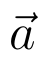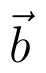Select Page

# MCQ in English Vector Algebra Solutions for CBSE Maths 12 Science

MCQ in English Vector Algebra Solutions for CBSE Maths 12 Science to enable students to get Solutions in a narrative video format for the specific question.

Expert Teacher provides MCQ Vector Algebra Solutions for CBSE Maths 12 Science through Video Solutions in English language. This video solution will be useful for students to understand how to write an answer in exam in order to score more marks. This teacher uses a narrative style for a question from Vector Algebra not only to explain the proper method of answering question, but deriving right answer too.

Please find the question below and view the Solution in a narrative video format.

Question:

Solution Video in English:

You can select video Solutions from other languages also. Please check Solutions in ( Hindi )

## Similar Questions from CBSE, 12th Science, Maths, Vector Algebra

Question 1 :  P and Q are two points with position vectorsandrespectively. Write the position vector of a point R which divides the line segment PQ externally in the ratio 2:1.  (View Answer Video)

Question 2 : Find |a| and |b|, if (a + b).(a - b) = 8 and |a| = 8|b|. (View Answer Video)

Question 3 : Find a unit vector parallel to the sum of vectorsand(View Answer Video)

Question 4 :  Ifanddenote the position vectors of points A and B respectively and C is a point on AB such that AC = 2 CB, then write the position vector of C.  (View Answer Video)

Question 5 : Find the angle between the vectorsand(View Answer Video)

### Integrals

Question 1 :(View Answer Video)

Question 2 :

Evaluate the following definite integral :(View Answer Video)

Question 3 : Find. (View Answer Video)

Question 4 : Evaluate :. (View Answer Video)

Question 5 : Evaluate :(View Answer Video)

### Inverse Trigonometric Functions

Question 1 : Write the principal value of(View Answer Video)

Question 2 : Solve for(View Answer Video)

Question 3 : Find the principal value of sin-1. (View Answer Video)

Question 4 : Solve the equation :(View Answer Video)

Question 5 : Write in the simplest form:(View Answer Video)

### Differential Equations

Question 1 : Write the sum of the order and degree of the differential equation(View Answer Video)

Question 2 : Write the degree of the differential equation :(View Answer Video)

Question 3 : Write the degree of the differential equation(View Answer Video)

Question 4 : Solve the following differential equation :(View Answer Video)

Question 5 : Find the particular solution of the differential equation :when x = 1,(View Answer Video)